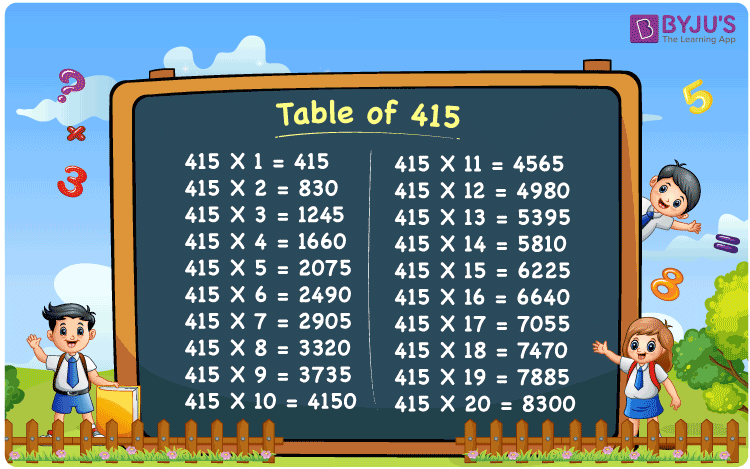Checkout JEE MAINS 2022 Question Paper Analysis : Checkout JEE MAINS 2022 Question Paper Analysis :

# Table of 415

The Table of 415 is crucial for the students who aspire to score remarkable results in their examination. For instance, 415 times 6 using repetitive addition can be written as 415 + 415 + 415 + 415 + 415 + 415 = 2490. In the table of 415, 415 × 6 = 2490. Students can find the multiplication of 415 till 20 here. The multiplication table of 415 is provided in PDF format which can be downloaded for free by clicking on the link available below.

## Table of 415 Chart## What is the 415 Times Table?

Memorizing the multiplication table of 415 is important for the students in their elementary education. We know that 415 times 8 using repetitive addition can be written as 415 + 415 + 415 + 415 + 415 + 415 + 415 + 415 = 3320. In the multiplication table of 415, 415 × 8 = 3320. Once this process is mastered by the students, they will be able to answer all the questions asked in their final exams.

 415 × 1 = 415 415 415 × 2 = 830 415 + 415 = 830 415 × 3 = 1245 415 + 415 + 415 = 1245 415 × 4 = 1660 415 + 415 + 415 + 415 = 1660 415 × 5 = 2075 415 + 415 + 415 + 415 + 415 = 2075 415 × 6 = 2490 415 + 415 + 415 + 415 + 415 + 415 = 2490 415 × 7 = 2905 415 + 415 + 415 + 415 + 415 + 415 + 415 = 2905 415 × 8 = 3320 415 + 415 + 415 + 415 + 415 + 415 + 415 + 415 = 3320 415 × 9 = 3735 415 + 415 + 415 + 415 + 415 + 415 + 415 + 415 + 415 = 3735 415 × 10 = 4150 415 + 415 + 415 + 415 + 415 + 415 + 415 + 415 + 415 + 415 = 4150

## Multiplication Table of 415

The Table of 415 has to be memorized thoroughly by the students to get a stronghold on the Mathematical calculations. After learning the multiplication tables on a daily basis, students will be able to answer the question based on multiples of 415 instantly. The objective of designing the table of 415 is to help students get a grip of the concepts without any difficulty.

 415 × 1 = 415 415 × 2 = 830 415 × 3 = 1245 415 × 4 = 1660 415 × 5 = 2075 415 × 6 = 2490 415 × 7 = 2905 415 × 8 = 3320 415 × 9 = 3735 415 × 10 = 4150 415 × 11 = 4565 415 × 12 = 4980 415 × 13 = 5395 415 × 14 = 5810 415 × 15 = 6225 415 × 16 = 6640 415 × 17 = 7055 415 × 18 = 7470 415 × 19 = 7885 415 × 20 = 8300

## Solved Example on the Table of 415

Q.1: 14 workers were appointed at a company in a day. How many workers will be appointed at the company in 415 days?

Solution: Given,

Number of workers appointed at a company in a day = 14

In 415 days number of workers appoints at the company = 415 x 14

= 6225

## Frequently Asked Questions on the Table of 415

### Evaluate 415 times 13.

From the table of 415, 415 multiplied by 13 is 415 x 13 = 5395.

### How many times should 415 be multiplied to get 4150?

In the table of 415, 415 x 10 = 4150. Hence, 415 should be multiplied by 10 to get 4150.

### Find 415 times 15 plus 1 minus 10.

Using the table of 415, 415 times 15 plus 1 minus 10 can be written as 415 x (15 + 1 – 10) = 415 x 6 = 2490